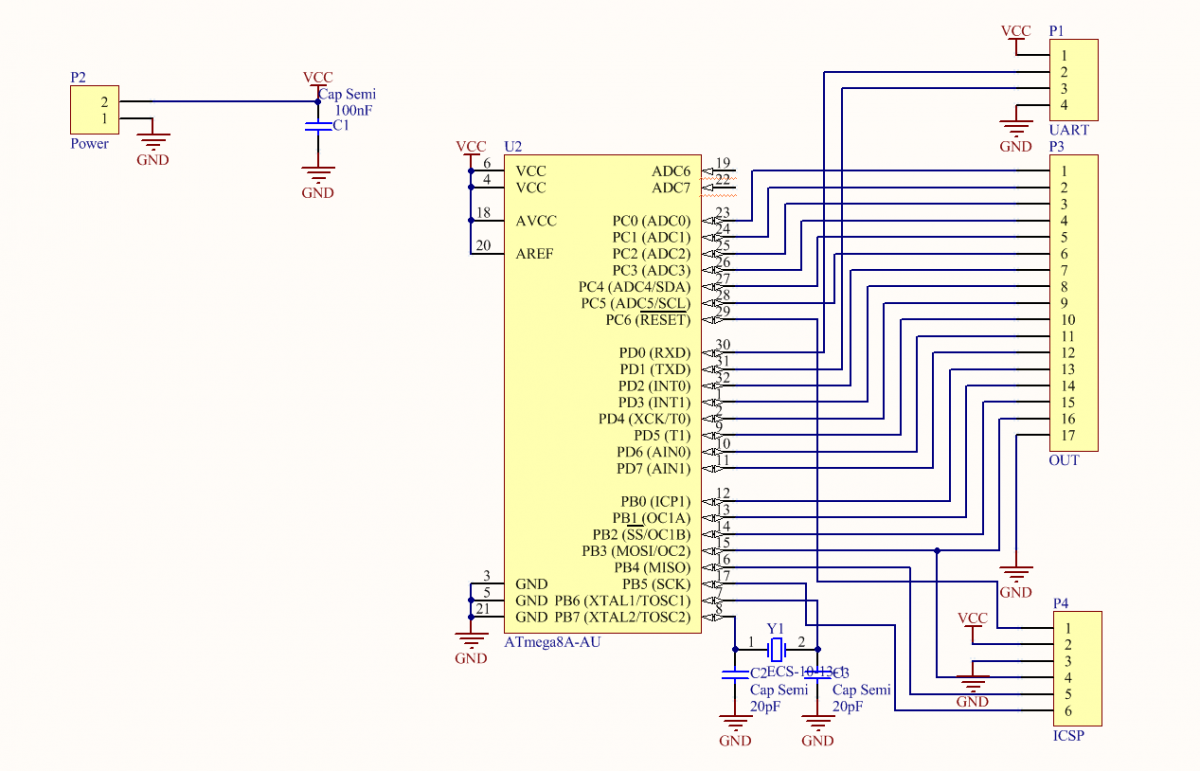# A MIDI Player on Eight Floppy Drives

DIY

Some times ago, I watched a video about singing floppy drives and a heap of written off computers, and decided to make something of the kind.Make it on Arduino like the rest? Seriously? As they say, f*ck the system! I decided to make it on Atmega8A, as that’s what I had at hand. I also decided that wires were unnecessary, and found HC-05.

Let’s go!

## The Circuit

As usual, the first stage is the circuit. No problem with that.It is simple, without unnecessary bells and whistles.

## The Board

It was no problem to make it with the help of the photoresist. Scattered everything in 1o minutes and produced it in an hour.## The Code

Now, the fun part begins — programming. To save some time, I decided to write in C. But I made the same mistake at the very beginning.

It was about controlling floppy drives. As you know, if Direct pin = 0, and we give impulses to Step, a floppy drive steps forward, and if Direct pin = 1, it makes a step back. But a floppy drive has only 80 steps. What can we do about that? The solution is simple. A floppy drive can make half a step if we change the state of Step, rather than pulsing it. It’s better to do it this way:

`Step ^= (1<<pin);` First problem solved! Now, let’s implement the control function.

``````void output(int drive) { // drive – the Floppy drive number 1 - 8
int position = drive - 1;
if(currentPosition[position] >= max)direct |= 1 << position;
if(currentPosition[position] <= 0)direct &= ~(1<<position); // set it to zero, if we are at 0
if(direct & (1<<position)) { //check the state
back(drive); //make a step back
currentPosition[position]--; //decrease the position of the current floppy drive
}else{
forward(drive); //step forward
currentPosition[position]++; //Increase it
}
}
``````

Done! Now, let’s deal with timers. Set the timer to the CTC mode with the division by 8 and allow interrupt by Output Compare (hereinafter OC). Put 40 in the OCR. An OC event occurs approximately in 40 µs. The interrupt handler is quite large.

``````void Action(){
if(fr1 != 0){
currentTick++;
if(currentTick >= fr1){ //fr* - periods of channels in µs
output(1);
currentTick=0;
}
};
if(fr2 != 0){
currentTick++;
if(currentTick >= fr2){
currentTick=0;
output(2);
}
};
if(fr3 != 0){
currentTick++;
if(currentTick >= fr3){
output(3);
currentTick=0;
}
};
if(fr4 != 0){
currentTick++;
if(currentTick >= fr4){
currentTick=0;
output(4);
}
};
if(fr5 != 0){
currentTick++;
if(currentTick >= fr5){
currentTick=0;
output(5);
}
};
if(fr6 != 0){
currentTick++;
if(currentTick >= fr6){
currentTick=0;
output(6);
}
};
if(fr7 != 0){
currentTick++;
if(currentTick >= fr7){
currentTick=0;
output(7);
}
};
if(fr8 != 0){
currentTick++;
if(currentTick >= fr8){
currentTick=0;
output(8);
}
};
}
ISR(TIMER2_COMP_vect){
Action();
}
``````

Now, the connection with the computer. Here we proceed as follows. Pass a string with a macro in the header (C/F) -> the body (number) -> the end of the package (‘;’). First, processing by the state machine:

``````void parse(){
char data = UDR;
switch(data){
case 'C': tmp_int = 0; SM= data; break;   // С – it’s the channel macro
case 'F': tmp_int = 0; SM= data; break;   // F - frequencies
case '0':
case '1':
case '2':
case '3':
case '4':
case '5':
case '6':
case '7':
case '8':
case '9': tmp_int = tmp_int * 10 + (data -'0'); break;
case ';': switch(SM){
case 'C': channel=tmp_int; break;
case 'F': freq = tmp_int; break;
}; play(freq, channel); break;  //in play, we recount the period, taking into account the clock frequency, then write it to fr*
case 'R' : resetAll(); break;
default: break;
}
}
``````

Second, we accept only in the Idle state:

`while ( UCSRA & (1<<RXC))parse();` — Done?

— No! How do we control it?

I didn’t feel like writing the control program from scratch, so I added functionality to HETC control terminal. Its interface is quite simple, so I’m not going to write much. The only thing is to select the Floppy drive and the COM-port of the device, then Connect.

Yes, you can do it without the Bluetooth, it’s just the way I see it.

Select the Midi file and….

## Source Code